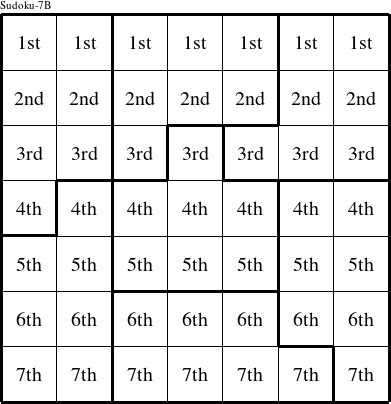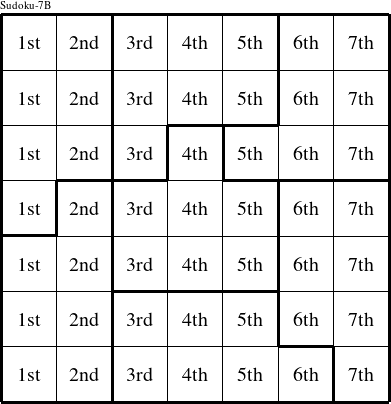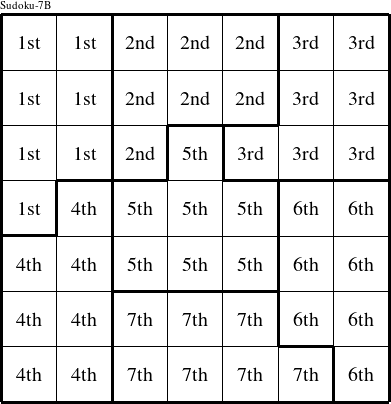#### Printable version

Detailed instructions for working a group puzzle of type 'Sudoku-7B'

The 7 elements in each group of this type of puzzle are: 1, 2, 3, 4, 5, 6, and 7.

Every group in the puzzle must contain exactly this set of elements.

####In a Sudoku-7B puzzle, each row is a group numbered as shown in the figure above.

####In a Sudoku-7B puzzle, each column is a group numbered as shown in the figure above.

####In a Sudoku-7B puzzle, each septomino is a group numbered as shown in the figure above.